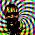## Monday, January 5, 2009

### How to Speed Up Octave

Speed seems like a continuing theme on the Octave mailing lists, a recent thread on the list of "comparing the executive speed with Matlab" demonstrates that there really is very little speed overhead when you use Octave smartly. If you read down far enough, it also demonstrates that the Intel Fortran compiler is awesome!

There are four basic methods of speeding up a chunk of Octave code (in order of increasing speed):
1. Allocate memory smartly (using for loops)

2. Vectorize your code (avoiding for loops)

3. Use sparse matrix operators in place of for loops you can't vectorize

4. Write a compiled function in C/C++/Fortran to call from Octave

The first three options keep you in pure Octave. The fourth option, while requiring you to use a compiled language is really not too bad because you can use all of the types (vectors, matrices, etc) defined in the Octave libraries to write your function. That's almost as easy as doing it in Octave.

### Speeding Up For Loops

This one really amounts to not being foolish. The only way to speed up for loops is to make sure you allocate all of your memory (using zeros() for instance) before you enter the for loop. The Octave Manual covers this topic here. The better thing to do is vectorize your code and avoid for loops all together.

### Vectorizing

What you really want to do is cast your calculation as a series of vector and matrix operations, or use the built-in (usually compiled) functions that operate directly on vectors. The post Is Octave Slow? demonstrates the sorts of speed up you can get when you get rid of for loops.

### Sparse Matrices for Data Dependancy

What about for loops you can't vectorize? The ones where the result at iteration n depends on what you calculated in iteration n-1, n-2, etc. You can define a sparse matrix that represents your for loop and then do a sparse matrix solve. This is much faster because you are now using compiled functions. The drawback is that you are trading some increase (sometimes substantial) in memory usage to buy this speed up.

### Compile It

The manual has a good intro on using mkoctfile to compile your function and make it callable from Octave. Probably the most useful thing is that you can use the Octave types, so you don't have to code up your own matrix or vector type. At this point the speed really has little to do with Octave and everything to do with the quality of the compiler you use and how efficiently you code up your calculation.

### Conclusion

The real power of Octave isn't that you can get your calculations to be nearly as fast as if you wrote them all in a compiled language. It's that you can prototype a calculation quickly and make sure that it is correct, and then there is a fairly well defined path to follow to speed up your correct calculation.

#### 1 comment:

1.I got this request for help on speeding up a moving average calculation by email recently.

> The original problem is
> for j=2:length(data)
> a(j) = a(j-1) + alpha*(data(j)-a(j-1));
> endfor
> Now after creating A as a sparse matrix, given the original above,
> what is "b" in your equation "x = A\b"?
>
The way I do it is to think of each iteration of the loop as an equation (see this post).

Writing out your loop I get a (alpha - 1) on the sub-diagonal, and a 1 on the main diagonal. I get an alpha*d(j) on the right-hand side, so that's your b.